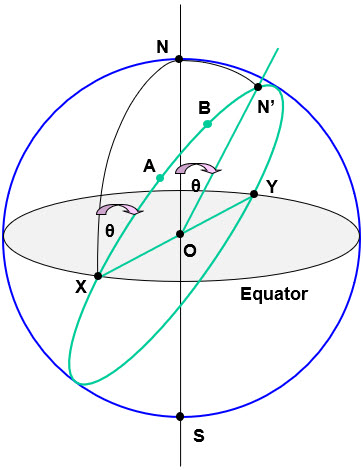# Globe Geometry

The geometry of 2D structures on a sheet of paper is reasonably familiar.  There is a similar characteristic geometry for a sphere, such as the globe or earth.  It has certain key features and behaviour.

A key feature of the globe is that it has an axis of rotation with North and South poles.  This is shown between the poles N & S in the diagram over.  The plane through the centre of the globe O perpendicular to this axis of rotation defines the Equator.

The basic element of 2D geometry is a line.  This is the shortest and straightest route between two points.  The equivalent element on a globe is an arc of a great circle.Take any two points on the surface of a globe, such as A & B in the diagram over.  The shortest and straightest route between these is an arc of a great circle.  This arc is continued right around the globe to illustrate the great circle in question.  This is shown in green.

All great circles have their centre at the centre of the globe O.  This is the key feature that defines a great circle.  It also means that points that share the same great circle also share the same connection to the center or origin O.

Every great circle crosses the equator at two points X & Y exactly opposite each other as shown.  Any great circle divides the equator in half and in turn is divided in half by the equator.  The mid way point along a great circle between the equator points X & Y defines the equivalent of a pole N’ on the northern arc and equivalently S’ on the southern arc.  N’ is also the point of closest approach to the N pole on the great circle.

We can join this pole N’ to the center O to get the equivalent of a vertical or polar axis N’ – O – S’ for the great circle in question.  The angle between the globe polar axis N – O and the great circle polar axis N’ – O, shown as θ, is a key feature of the great circle.  This is its angle of inclination from the polar axis or its angle of declination, i.e. how much it deviates from the pole.  For example, all the meridians of longitude, e.g. X – N, pass through the poles and have 0 declination, while the equator has a 90° angle of declination.

This angle of declination shows up in two locations on the surface of the globe.  N’ for any great circle is its point of closest approach to the N pole, i.e. it is the point of highest latitude.  The difference in latitude between the N and N’, i.e. N – N’, is a direct measure of the angle of declination θ.  A great circle will form an angle with the equator at its crossing points, e.g. X.  90° minus this angle is also a direct measure of the declination angle θ.  This is also the angle between the meridian through the crossing point, X – N, and the great circle at X, as shown in the diagram.